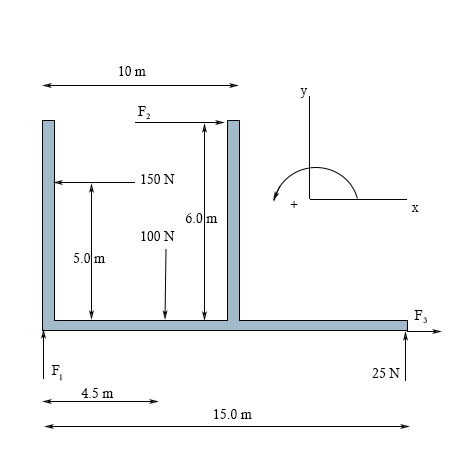# A rigid structure is shown below. Find the following: a) Use \Sigma M = 0 around F_1 to find F_2...

## Question:

A rigid structure is shown below. Find the following:

a) Use {eq}\Sigma M = 0 {/eq} around {eq}F_1 {/eq} to find {eq}F_2 {/eq} (N),

b) Use {eq}\Sigma F_2 = 0 {/eq} to find the value of {eq}F_3 {/eq} (N)

c) Use {eq}\Sigma F_3 = 0 {/eq} to find the value of {eq}F_1 {/eq} (N)## Static equilibrium:

A rigid body acted upon by different external forces is said to be in static equilibrium if all the forces and moments are balanced or their algebraic sum is zero, like:

{eq}\sum M=0\\ \sum F_{x}=0\\ \sum F_{y}=0\\ {/eq}

## Answer and Explanation:

(a)

Applying, {eq}\sum M_{F_{1}}=0\\ \Rightarrow (F_{2}\times 6)-(150\times 5)+(100\times 4.5)-(25\times 15)=0\\ \Rightarrow F_{2}=112.5\:...

See full answer below.

Become a Study.com member to unlock this answer! Create your account

#### Learn more about this topic:Statics Problem-Solving Methods & Applications

from UExcel Physics: Study Guide & Test Prep

Chapter 8 / Lesson 3
6.4K